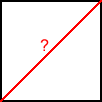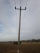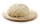# Square diagonal

Calculate the length of the square diagonal if the perimeter is 476 cm.

Result

d =  168.29 cm

#### Solution:

$o=476 \ \text{cm} \ \\ \ \\ o=4a \ \\ \ \\ a=o/4=476/4=119 \ \text{cm} \ \\ \ \\ d^2=a^2 + a^2=119^2 + 119^2=28322 \ \\ \ \\ d=\sqrt{ 2 } \cdot \ a=\sqrt{ 2 } \cdot \ 119 \doteq 119 \ \sqrt{ 2 } \doteq 168.2914 \doteq 168.29 \ \text{cm}$Our examples were largely sent or created by pupils and students themselves. Therefore, we would be pleased if you could send us any errors you found, spelling mistakes, or rephasing the example. Thank you!

Leave us a comment of this math problem and its solution (i.e. if it is still somewhat unclear...):Be the first to comment!Tips to related online calculators
Pythagorean theorem is the base for the right triangle calculator.

#### You need to know the following knowledge to solve this word math problem:

We encourage you to watch this tutorial video on this math problem:

## Next similar math problems:

1. Height of pyramidThe pyramid ABCDV has edge lengths: AB = 4, AV = 7. What is its height?
2. Circle and squareAn ABCD square with a side length of 100 mm is given. Calculate the radius of the circle that passes through the vertices B, C and the center of the side AD.The surface of the regular quadrilateral prism is 8800 cm2, the base edge is 20 cm long. Calculate the volume of the prism
4. What isWhat is the annual percentage increase in the city when the population has tripled in 20 years?
5. Side lengthsIn the triangle ABC, the height to the side a is 6cm. The height to side b is equal to 9 cm. Side "a" is 4 cm longer than side "b". Calculate the side lengths a, b.
6. TV towerCalculate the height of the television tower if an observer standing 430 m from the base of the tower sees the peak at an altitude angle of 23°?Calculate the surface of a quadrilateral pyramid, which has a rectangular base with dimensions a = 8 cm, b = 6 cm and height H = 10 cm.
8. The circumferenceThe circumference and width of the rectangle are in a ratio of 5: 1. its area is 216cm2. What is its length?
9. Cutting coneA cone with a base radius of 10 cm and a height of 12 cm is given. At what height above the base should we divide it by a section parallel to the base so that the volumes of the two resulting bodies are the same? Express the result in cm.
10. Observation towerFrom the observation tower at a height of 105 m above sea level, the ship is aimed at a depth angle of 1° 49´. How far is the ship from the base of the tower?The ladder touch on a wall at a height of 7.5 m. The angle of the inclination of the ladder is 76°. How far is the lower end of the ladder from the wall?The bases of two adjacent telegraph poles have a height difference of 10.5 m. How long do the wires connect the two poles if the slope is 39° 30´?The dough contains water, flour, and sugar. Water and flour in a ratio of 2: 3, flour, and sugar in a ratio of 2: 1. Determine the ratio of all three components of the dough.How long will it take to save € 9,000 by depositing € 200 at the beginning of each year at 2% interest?The age of father and son is in the ratio 10: 3. The age of the father and daughter is in ratio 5: 2. How old are a father and a son if the daughter is 20?The observer sees a straight fence 60 m long at a viewing angle of 30°. It is 102 m away from one end of the enclosure. How far is the observer from the other end of the enclosure?The number 72 increase by 25%. By how much % will you have to reduce the number you created to get the number 72 again?